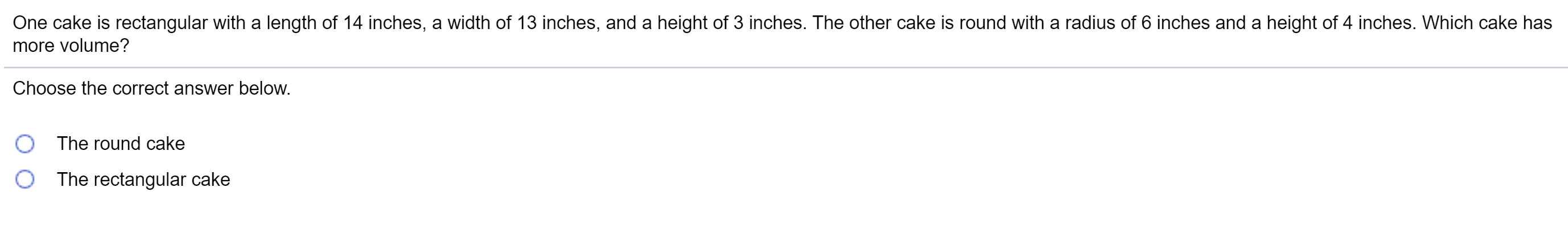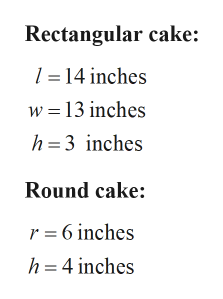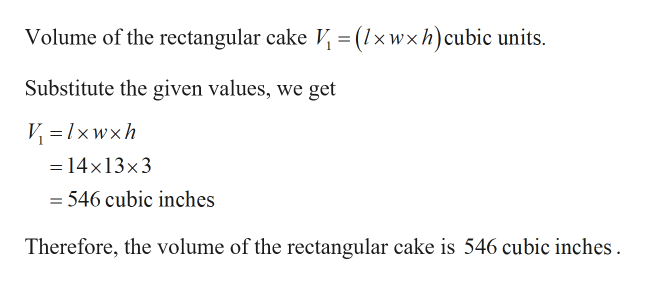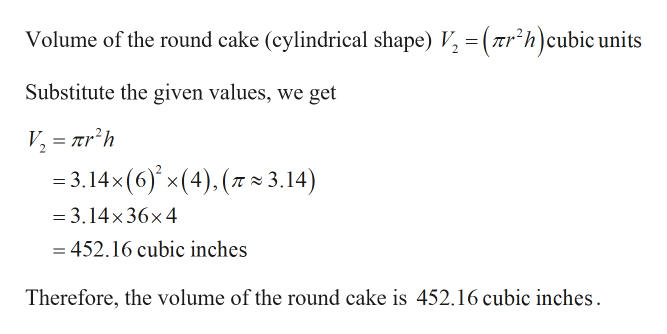# One cake is rectangular with a length of 14 inches, a width of 13 inches, and a height of 3 inches. The other cake is round with a radius of 6 inches and a height of 4 inches. Which cake hasmore volume?Choose the correct answer below.The round cakeThe rectangular cake

Question
2 viewshelp_outlineImage TranscriptioncloseOne cake is rectangular with a length of 14 inches, a width of 13 inches, and a height of 3 inches. The other cake is round with a radius of 6 inches and a height of 4 inches. Which cake has more volume? Choose the correct answer below. The round cake The rectangular cake fullscreen
check_circle

Step 1

Given:help_outlineImage TranscriptioncloseRectangular cake: 1 = 14 inches w = 13 inches h= 3 inches Round cake: r = 6 inches h = 4 inches fullscreen
Step 2

Calculation:

To find a cake which has more volume:help_outlineImage TranscriptioncloseVolume of the rectangular cake V, = (1×wxh)cubic units. Substitute the given values, we get V = 1xwxh = 14x13×3 = 546 cubic inches Therefore, the volume of the rectangular cake is 546 cubic inches. fullscreen
Step 3help_outlineImage TranscriptioncloseVolume of the round cake (cylindrical shape) V, = ( Tr*h)cubic units Substitute the given values, we get V, = ar’h = 3.14x(6) x(4), (7 × 3.14) = 3.14x 36x4 = 452.16 cubic inches Therefore, the volume of the round cake is 452.16 cubic inches. fullscreen

### Want to see the full answer?

See Solution

#### Want to see this answer and more?

Solutions are written by subject experts who are available 24/7. Questions are typically answered within 1 hour.*

See Solution
*Response times may vary by subject and question.
Tagged in

### Other